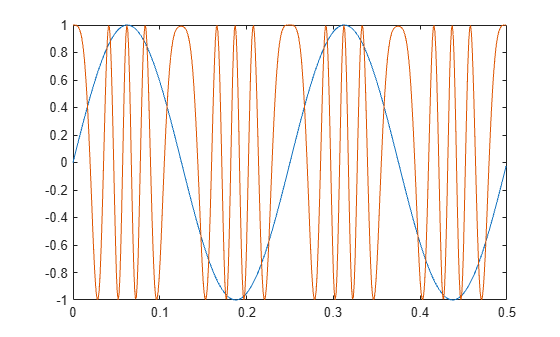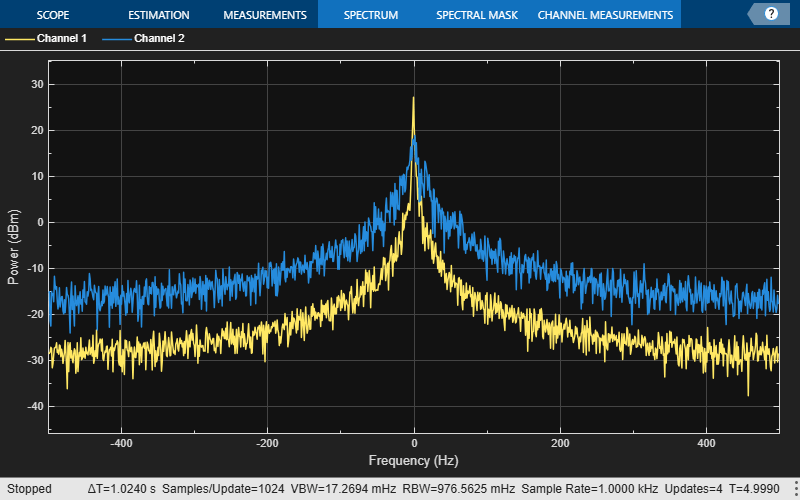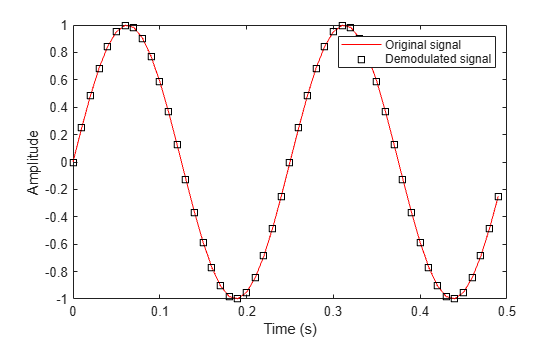# comm.FMModulator

Modulate signal using FM method

## Description

The `comm.FMModulator` System object™ applies baseband frequency modulation to a signal. For more information, see Algorithms.

To modulate a signal using the FM method:

1. Create the `comm.FMModulator` object and set its properties.

2. Call the object with arguments, as if it were a function.

To learn more about how System objects work, see What Are System Objects?

## Creation

### Syntax

``fmmodulator = comm.FMModulator``
``fmmodulator = comm.FMModulator(Name,Value)``
``fmmodulator = comm.FMModulator(fmdemodulator)``

### Description

````fmmodulator = comm.FMModulator` creates an FM modulator System object.```

example

````fmmodulator = comm.FMModulator(Name,Value)` sets properties using one or more name-value arguments. For example, `'SampleRate,400e3'` specifies a sample rate of 400 kHz.```

example

````fmmodulator = comm.FMModulator(fmdemodulator)` sets properties based on the configuration of the input `comm.FMDemodulator` System object, `fmdemodulator`.```

## Properties

expand all

Unless otherwise indicated, properties are nontunable, which means you cannot change their values after calling the object. Objects lock when you call them, and the `release` function unlocks them.

If a property is tunable, you can change its value at any time.

For more information on changing property values, see System Design in MATLAB Using System Objects.

Sample rate of the input signal in Hz, specified as a positive scalar. This property specifies the sample rate at the output of a modulator or at the input of a demodulator. The sample rate must be greater than twice the frequency deviation (that is, `SampleRate` > 2×`FrequencyDeviation`).

Data Types: `double`

Peak deviation of the output signal frequency in Hz, specified a positive scalar. The frequency deviation must be less than half the sample rate (that is, `FrequencyDeviation` < `SampleRate`/2).

The system bandwidth is BT = 2×(`FrequencyDeviation` + BM), where BM is the message bandwidth in Hz. For more information, see Algorithms.

Data Types: `double`

## Usage

### Syntax

``outsig = fmmodulator(insig)``

### Description

example

````outsig = fmmodulator(insig)` modulates the input message signal and outputs a baseband FM signal.```

### Input Arguments

expand all

Input signal, specified as a scalar or column vector.

Data Types: `double` | `single` | `fi`
Complex Number Support: Yes

### Output Arguments

expand all

Modulated baseband FM signal, returned as a scalar or column vector with complex values. The output signal has the same data type and size as the input `insig`.

## Object Functions

To use an object function, specify the System object as the first input argument. For example, to release system resources of a System object named `obj`, use this syntax:

`release(obj)`

expand all

 `step` Run System object algorithm `release` Release resources and allow changes to System object property values and input characteristics `reset` Reset internal states of System object

## Examples

collapse all

Apply baseband modulation to a sine wave. Plot the sine wave and the modulated signals.

Set parameters for the example.

```fs = 1e3; % Sample rate (Hz) ts = 1/fs; % Sample period (s) fd = 50; % Frequency deviation (Hz)```

Create a sinusoidal signal with a duration of 0.5 s and frequency of 4 Hz.

```t = (0:ts:0.5-ts)'; x = sin(2*pi*4*t);```

Create an FM modulator System object™, setting the sample rate and frequency deviation.

```fmmodulator = comm.FMModulator( ... 'SampleRate',fs, ... 'FrequencyDeviation',fd);```

FM-modulate the signal and plot its real part. The frequency of the modulated signal changes with the amplitude of the input signal.

```y = fmmodulator(x); plot(t,[x real(y)])```Apply baseband FM modulation to a white Gaussian noise source and plot the spectrum of the modulated signal.

Initialize parameters for the example.

```fs = 1e3; % Sample rate (Hz) ts = 1/fs; % Sample period (s) fd = 10; % Frequency deviation (Hz)```

Create a white Gaussian noise source with a duration of 5 seconds.

```t = (0:ts:5-ts)'; x = wgn(length(t),1,0);```

Create two FM modulator System objects, setting the sample rate and frequency deviation. Set the frequency deviation of the second FM modulator object five times higher than the first FM modulator.

```fmmod1 = comm.FMModulator( ... SampleRate=fs, ... FrequencyDeviation=fd); fmmod2 = comm.FMModulator( ... SampleRate=fs, ... FrequencyDeviation=5*fd);```

Use the FM modulators to apply FM modulation to the signal `x`.

```y1 = fmmod1(x); y2 = fmmod2(x);```

Plot the spectra of the two modulated signals. The larger frequency deviation associated with channel 2 results in a noise level that is 10 dB higher than the first channel.

```specanalyzer = spectrumAnalyzer(SampleRate=fs,ShowLegend=true); specanalyzer([y1 y2]) release(specanalyzer)```Modulate and demodulate a sinusoidal signal. Plot the demodulated signal and compare it to the original signal.

Initialize parameters for the example.

```fs = 100; % Sample rate (Hz) ts = 1/fs; % Sample period (s) fd = 25; % Frequency deviation (Hz)```

Create a sinusoidal signal with a duration of 0.5 s and frequency of 4 Hz.

```t = (0:ts:0.5-ts)'; x = sin(2*pi*4*t);```

Create an FM modulator System object™, setting the sample rate and frequency deviation. Then, create an FM demodulator System object, using the FM modulator configuration to set the demodulator properties.

```fmmodulator = comm.FMModulator( ... 'SampleRate',fs, ... 'FrequencyDeviation',fd); fmdemodulator = comm.FMDemodulator(fmmodulator);```

FM-modulate the signal and plot the real component of the complex signal. The frequency of the modulated signal changes with the amplitude of the input signal.

```y = fmmodulator(x); plot(t,[x real(y)]) title('Input Sinusoid and FM-Modulated Signals') xlabel('Time (seconds)'); ylabel('Amplitude') legend('Input signal','Modulated signal (real component)')```Demodulate the FM-modulated signal.

`z = fmdemodulator(y);`

Plot the original and demodulated signals. The demodulator output signal exactly aligns with the original signal.

```plot(t,x,'r',t,z,'ks') legend('Original signal','Demodulated signal') xlabel('Time (s)') ylabel('Amplitude')```## Algorithms

A frequency-modulated passband signal, Y(t), is given as

`$Y\left(t\right)=A\mathrm{cos}\left(2\pi {f}_{\text{c}}t+2\pi {f}_{\text{Δ}}{\int }_{0}^{t}x\left(\text{τ}\right)d\text{τ}\right)\text{\hspace{0.17em}},$`

where:

• A is the carrier amplitude.

• fc is the carrier frequency.

• x(τ) is the baseband input signal.

• fΔ is the frequency deviation in Hz.

The frequency deviation is the maximum shift from fc in one direction, assuming |x(τ)| ≤ 1.

A baseband FM signal can be derived from the passband representation by downconverting the passband signal by fc such that

`$\begin{array}{c}{y}_{\text{s}}\left(t\right)=Y\left(t\right){e}^{-j2\pi {f}_{\text{c}}t}=\frac{A}{2}\left[{e}^{j\left(2\text{π}{f}_{\text{c}}t+2\text{π}{f}_{\text{Δ}}{\int }_{0}^{t}x\left(\text{τ}\right)d\text{τ}\right)}+{e}^{-j\left(2\text{π}{f}_{\text{c}}t+2\text{π}{f}_{\text{Δ}}{\int }_{0}^{t}x\left(\text{τ}\right)d\text{τ}\right)}\right]{e}^{-j2\text{π}{f}_{\text{c}}t}\\ =\frac{A}{2}\left[{e}^{j2\text{π}{f}_{\text{Δ}}{\int }_{0}^{t}x\left(\text{τ}\right)d\text{τ}}+{e}^{-j4\text{π}{f}_{\text{c}}t-j2\text{π}{f}_{\text{Δ}}{\int }_{0}^{t}x\left(\text{τ}\right)d\text{τ}}\right]\text{\hspace{0.17em}}.\end{array}$`

Removing the component at -2fc from yS(t) leaves the baseband signal representation, y(t), which is given as

`$y\left(t\right)=\frac{A}{2}{e}^{j2\pi {f}_{\Delta }{\int }_{0}^{t}x\left(\tau \right)d\tau }.$`

The expression for y(t) can be rewritten as $y\left(t\right)=\frac{A}{2}{e}^{j\varphi \left(t\right)}$, where $\varphi \left(t\right)=2\text{π}{f}_{\Delta }{\int }_{0}^{t}x\left(\tau \right)d\tau$. Expressing y(t) this way implies that the input signal is a scaled version of the derivative of the phase, ϕ(t).

To recover the input signal from y(t), use a baseband delay demodulator, as this figure shows.Subtracting a delayed and conjugated copy of the received signal from the signal itself results in this equation.

`$w\left(t\right)=\frac{{A}^{2}}{4}{e}^{j\varphi \left(t\right)}{e}^{-j\varphi \left(t-T\right)}=\frac{{A}^{2}}{4}{e}^{j\left[\varphi \left(t\right)-\varphi \left(t-T\right)\right]}\text{\hspace{0.17em}},$`

where T is the sample period. In discrete terms,

The signal vn is the approximate derivative of ϕn such that vnxn.

 Hatai, I., and I. Chakrabarti. “A New High-Performance Digital FM Modulator and Demodulator for Software-Defined Radio and Its FPGA Implementation.” International Journal of Reconfigurable Computing (December 25, 2011): 1–10. https://doi.org/10.1155/2011/342532.

 Taub, H., and D. Schilling. Principles of Communication Systems. McGraw-Hill Series in Electrical Engineering. New York: McGraw-Hill, 1971, pp. 142–155.